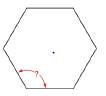Chapter 2.5, Problem 38E### Elementary Geometry for College St...

6th Edition
Daniel C. Alexander + 1 other
ISBN: 9781285195698

#### Solutions

Chapter
Section### Elementary Geometry for College St...

6th Edition
Daniel C. Alexander + 1 other
ISBN: 9781285195698
Textbook Problem
1 views

# The top surface of a picnic table is in the shape of a regular hexagon. What is the measure of the angle formed by two consecutive sides?To determine

To find:

The measure of the angle formed by two consecutive sides of a picnic table has the shape of a regular hexagon with 6 sides.

Explanation

Given:

The following figure shows the given picnic table.

The given clock is a regular polygon of 6 sides.

Formula used:

The formula to calculate the angle formed by two consecutive sides of an n sided regular polygon is given by,

I=(n2)180°n

Here, I denotes each interior angle.

Calculation:

Substitute 6 for n in the above mentioned formula to obtain the angle formed by two consecutive sides of a picnic table

### Still sussing out bartleby?

Check out a sample textbook solution.

See a sample solution

#### The Solution to Your Study Problems

Bartleby provides explanations to thousands of textbook problems written by our experts, many with advanced degrees!

Get Started

#### Sketch the graphs of the equations in Exercises 512. xy=2x3+1

Finite Mathematics and Applied Calculus (MindTap Course List)

#### Evaluate limx01+tanx1+sinxx3.

Single Variable Calculus: Early Transcendentals, Volume I

#### 4. Solve using the quadratic formula.

Mathematical Applications for the Management, Life, and Social Sciences